# We Helped With This JAVA Programming Homework: Have A Similar One?

SOLVEDCategory Programming JAVA Undergraduate Solved Assignments Java

## Short Assignment Requirements

I need you to do it as the extra credit part please. Also, provide a paragraph discussing the algorithm.Thanks

## Assignment Image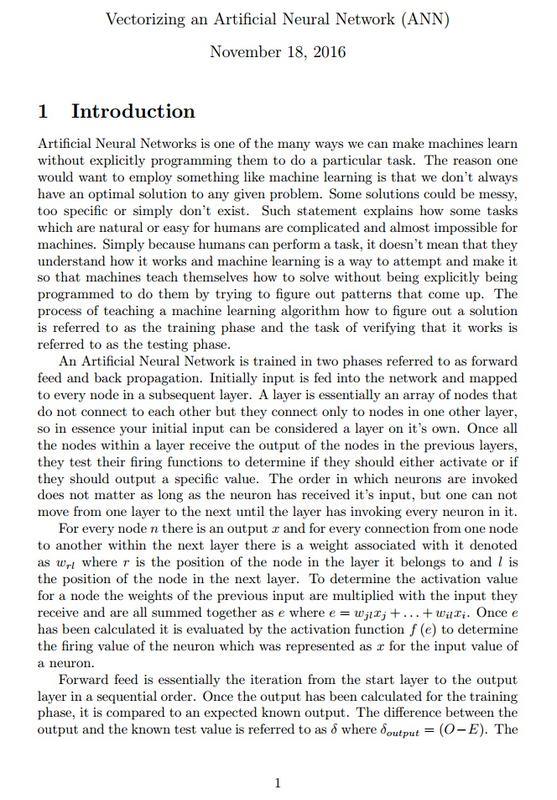Vectorizing an Artificial Neural Network (ANN) November 18, 2016 1 Introduction Artificial Neural Networks is one of the many ways we can make machines learn without explicitly programming them to do a particular task. The reason one would want to employ something like machine learning is that we don't always have an optimal solution to any given problem. Some solutions could be messy, too specific or simply don't exist. Such statement explains how some tasks which are natural or easy for humans are complicated and almost impossible for machines. Simply because humans can perform a task, it doesn't mean that they understand how it works and machine learning is a way to attempt and make it so that machines teach themselves how to solve without being explicitly being programmed to do them by trying to figure out patterns that come up. The process of teaching a machine learning algorithm how to figure out a solution is referred to as the training phase and the task of verifying that it works is referred to as the testing phase. An Artificial Neural Network is trained in two phases referred to as forward feed and back propagation. Initially input is fed into the network and mapped to every node in a subsequent layer. A layer is essentially an array of nodes that do not connect to each other but they connect only to nodes in one other layer, so in essence your initial input can be considered a layer on it's own. Once all the nodes within a layer receive the output of the nodes in the previous layers, they test their firing functions to determine if they should either activate or if they should output a specific value. The order in which neurons are invoked does not matter as long as the neuron has received it's input, but one can not move from one layer to the next until the layer has invoking every neuron in it. For every node n there is an output xr and for every connection from one node to another within the next layer there is a weight associated with it denoted as w, where r is the position of the node in the layer it belongs to and I is the position of the node in the next layer. To determine the activation value for a node the weights of the previous input are multiplied with the input they receive and are all summed together as e where e = wjxj+...+wili. Once e has been calculated it is evaluated by the activation function f (e) to determine the firing value of the neuron which was represented as a for the input value of a neuron. Forward feed is essentially the iteration from the start layer to the output layer in a sequential order. Once the output has been calculated for the training phase, it is compared to an expected known output. The difference between the output and the known test value is referred to as & where Soutput = (O-E). The 1

## Assignment Image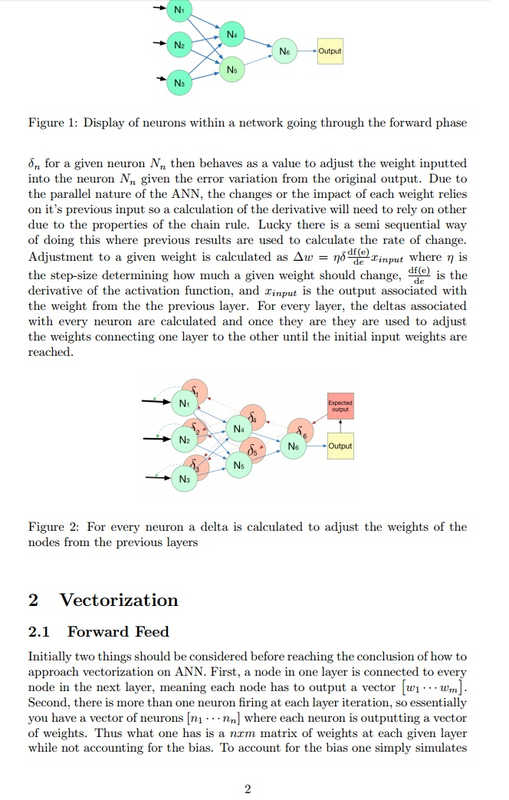N₁ N₂ N3 N₁ N4 N₂ N₁ Figure 1: Display of neurons within a network going through the forward phase N3 Sn for a given neuron N₁, then behaves as a value to adjust the weight inputted into the neuron N₁ given the error variation from the original output. Due to the parallel nature of the ANN, the changes or the impact of each weight relies on it's previous input so a calculation of the derivative will need to rely on other due to the properties of the chain rule. Lucky there is a semi sequential way of doing this where previous results are used to calculate the rate of change. Adjustment to a given weight is calculated as Aw = nod(e) input where n is the step-size determining how much a given weight should change, dife) is the derivative of the activation function, and input is the output associated with the weight from the the previous layer. For every layer, the deltas associated with every neuron are calculated and once they are they are used to adjust the weights connecting one layer to the other until the initial input weights are reached. N4 Ns N6 ds' Output se 2 No Expected output Output Figure 2: For every neuron a delta is calculated to adjust the weights of the nodes from the previous layers 2 Vectorization 2.1 Forward Feed Initially two things should be considered before reaching the conclusion of how to approach vectorization on ANN. First, a node in one layer is connected to every node in the next layer, meaning each node has to output a vector [w₁... wm]. Second, there is more than one neuron firing at each layer iteration, so essentially you have a vector of neurons [n₁nn] where each neuron is outputting a vector of weights. Thus what one has is a næm matrix of weights at each given layer while not accounting for the bias. To account for the bias one simply simulates

## Assignment Description

Project : ANN

For this lab, you will be tasked with building a neural network that takes in an a text file with a set of data and then trains the network on that said data. In addition to that, you will need to provide the user with command line interface which will prompt the user for an input and then provide an output.

Every node will need to have to use a nonlinear function which will work as it’s activation function a good example would be a sigmoid function.

Your network will need to have a condition which will make it stop. Preferably you want it to be so that you would stop when your error levels are bellow a specific desired value.

You can test this code by training the network to recolonize a logic gate.

Your user interface should look something like this:

Set the  number of hidden layers needed

> 2

Set the size of hidden layer 1

> 2

Set  the size of hidden layer 2

> 2

Creating  a 2-input layer, 2-hidden layer, 2-hidden layer, 1 output layer      ANN

Initializing weights values of the  neurons and bias node to random values between 1 and 10

Set the max number of loops before stopping. (In case the network is unable to converge)

> 900

Set the learning rate for the network

> 0.01

Setting maxEpochs to 900 learningRate to 0.01

Normalizing input

************

Training using BackPropgation

************

(ﾉ）ﾉ ┻━┻

ノ( º _ ºノ)

(ノಠ)ノ彡┻━┻

── ¯\_(ツ)

ノ( º _ ºノ)

┻━┻ (`Д´)ﾉ ┻━┻

************

Training Compete

************

Test an input:

> 0 0

0

Test an input:

> 1 1

1

## Assignment Description

Lab1

Matrix operations

August 30, 2016

### 1          About Multidimensional Arrays in Java

#### 1.1     Introduction

Recall from your previous lecture that an array is a container object or a collection object. Collections in Java (or any other language for that matter) work as objects that reference other objects thus keeping them in a ”collection”. In java, Arrays are collections with a fixed number of values that are ordered (indexed) and are usually a single fixed type (recall polymorphism). Multidimensional arrays are exactly that, except instead of representing just any objects they represent nested arrays. The arrays are nested in the same sense as nested loops consist of loops within one another. In other words, a 2D array is really just an array of arrays of a particular type. A 3D array is an array of arrays, of arrays of a particular type or an array of 2D arrays.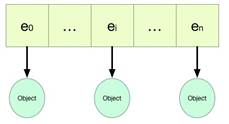Figure 1: In Java an Array is a collection of references which point to a specific type of object.

Review of how to use arrays in Java

int[] myIntarray = null; // an empty reference of type array myIntarray = new int; // an allocated array of size 10

// meaning 10 int values can be stored System.out.println(myIntarray.length);               // prints out 10

int[] myOtherIntArray = {0,1,2,3,4,5,6,7,8,9}; // Another way of creating

// a 1D array of the same size

//but with actual values

System.out.println(myOtherIntArray.length);                                  // prints out 10

#### 1.2     2D arrays

2D arrays are highly useful in most applications. They can easily be used to represent tables or matrices among many other things. As mentioned earlier, 2D arrays are arrays of specific arrays. The way they are represented is as such. Each entry of the outer array represents an array of it’s own being. Each one of those arrays can either host other arrays or simply host objects like a regular array.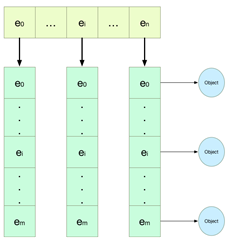Figure 2: A 2D array works by having an array reference multiple other arrays thus simulating a table or a matrix like representation even though that is not the case in memory

How to use 2D arrays in Java Consider the code bellow. The array objects myArrayofIntArrays2 and myArrayofIntArrays are arrays of size 5 hosting 5 different int arrays of size 2.

int[][] myArrayofIntArrays;                // reference a 2D array of type int myArrayofIntArrays = new int; // allocation 5 arrays of size 2

System.out.println(myArrayofIntArrays.length); // prints out 5

System.out.println(myArrayofIntArrays.length); // prints out 2

// Another way of doing the same thing but with values // Have you noticed how it is an array of arrays?

// This is called a Nested array initializer

// Because the arrays are nested within an array int[][] myArrayofIntArrays2 = {{1,2},{3,4},{5,6},{7,8},{9,10}};

System.out.println(myArrayofIntArrays2.length); // prints out 5 System.out.println(myArrayofIntArrays2.length); // prints out 2

System.out.println(Arrays.toString(myArrayofIntArrays2)); // prints out [1, 2]

#### 1.3      Jagged arrays

Arrays within an array don’t have to be of equal size. Remember that arrays are only reference holders and mutable as well (can change within memory). Jagged arrays are nested arrays with varying sizes. These are great for nonuniform data, but be careful not to reference an index that is out of bound. int [][] myJaggedArray = {{1},{2,3},{3,4,5}}

#### 1.4       Iterating through a 2D array

for loops To iterate through a 2D arrays, one can simply use a nested for loop. The process is simple, one goes through the outer array sequentially within the outer loop, and for the inner loop the programmer can simply iterate each array at that particular position.

int [][] myDoubleArray;

//...some code for (int n = 0; n < myDoubleArray.length; n += 1)

{ for (int m = 0; m < myDoubleArray[n].length; m += 1) {

}

}

for each loops are much more easier and simpler to write. One iterates through the array of arrays by taking in a single array each iteration. Once an array is gained, one can just do the same an iterate through it gaining the elements desired sequentially.

for(int[] myArray : myDoubleArray)

// for each array in my double array {

// for each element in my array for (int element : myArray) {

//iterates through each element

}

}

### 2       Matrix operation

This is not a math appreciation project, Matrix operations are used extensively in computation. As an engineer you would be introduced to them in the form of machine vision, improving system performance, and as systems of differential equations. As a programmer you will more than likely see them if you are part of a specific domain. For example, recommendation systems such as that used by Google and Amazon rely greatly on matrix operation so that they can provide the specific recommendations needed for multiple users. The same can be applied for other recommendation systems as well, however the scope for such topics is way beyond the class so just know that they can be used for things other than graphics. Long story short, you have to use matrices if you want to program in parallel or address networks (communication between systems especially). This project is just a way to introduce you to something that might be applicable to you in the future.

Matrix addition and subtraction are rather straightforward operations. The sum of matrix A and matrix B given that the two matrices are of size m × n is calculated as shown below. each element aij in matrix A where 1 ≤ i m and 1 ≤ j m is added to element bij in matrix B (aij + bij).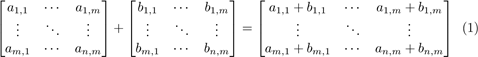The difference of matrix A and matrix B given that the two matrices are of size m × n is calculated as shown below. each element aij in matrix A where 1 ≤ i m and 1 ≤ j m is subtracted to element bij in matrix B.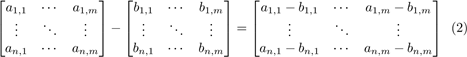#### 2.2      Multiplication

The product of two matrices (say A and B) is another matrix. For two matrices to be multiplied (AB) the rows of the first matrix must match the rows of the second matrix. The result matrix’s dimensions is the columns of the first matrix and the rows of the second matrix where each entry is a series of summation of each entry in the first matrix column multiplied by it’s transposed equivalent from the second matrix (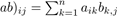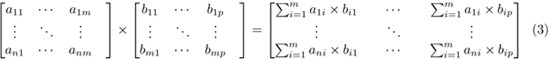### 3      Lab 1

For your first project you will be tasked with implementing an object to represent a matrix of integers. For the object, I used three different fields to represent it and make my life a little easier. A double array to represent the location of each element in the matrix and two integer values to represent the dimensions of the matrix (row, column). You are free to use as many fields as you need however it is advised to try and keep things clean and less cluttered.

3.1         What would your Main method consist of?

In your main class, you need to write an interface for the user to select the size of his/her desired matrices and the operation to be executed on the two matrices randomly generated. Have a text prompt the user to input the size of one or two of the parameters, such as the columns, the rows, or both at the same time. Once that is done, prompt the user to select an operation to use.

They can be numbered from 1 to 3 as such:

Select the operation to executed:

2.   Subtract3. Multiply

> 1

#### 3.2      Constructor

Write two different constructors for the Matrix class. The first constructor should initialize the matrix without any input parameter. The second constructor should take in an array as an input parameter and creates an equivalent matrix object with that particular array.

public Matrix(int rows, int columns) {

// Initialize the object’s fields (class variables)

// given the sizes provided as input parameters // then populate the matrix with random numbers populatematrix(-100, 100);

}

public Matrix(int [][] matrixArray) {

// transform this Array into a new matrix object

// remember Arrays are mutable so you might want to

// make a new copy of the input parameter before using it }

#### 3.3        populating the Matrix with randomly numbers

You will need to write a method to populate your ”Matrix” which is essentially an array encapsulated in the matrix object. The method needs to generate a number from a positives and negative range. The range should not be set in stone, rather it should be an input parameter to the method.

private void populatematrix(int min, int max)

{

Random randnum = new Random();

// Iterate through the array representing your matrix

// and assign it a random value within

// the given range for (// 0 to the number of rows)

{ for (// 0 to the number of columns)

{

// Put a random number within the given range

// min and max

}

}

}

You will need to impediment two methods for adding and subtracting ”matrices” together. The method should take in another ”matrix” and return the result as a ”matrix”.

{

// add two matrices of the same size // Hint: use 2 nested loops

return new Matrix(resultMatrixArray);

}

public Matrix subtract(Matrix otherMatrix)

{

// subtract two matrices of the same size // Hint: use 2 nested loops

return new Matrix(resultMatrixArray);

}

#### 3.5      Multiplication

Like the addition and subtraction methods you will need to also make a multiplication method. However unlike the addition and subtraction methods you can’t just multiply the equivalent positions in a matrix. The size of the matrix can vary depending on two input matrices and in some cases it could be illegal to multiply them both. Check for valid inputs, and be sure to add the additional inner loop which will be discussed in class.

public Matrix dotProduct (Matrix otherMatrix)

{

// implement a method which takes in another

// matrix as a parameter, and multiplies

// it by the matrix representation stored

// in the object it’s self

// hint: It is 3 nested loops

// the rows of the result matrix

// the columns of the result matrix

// and the calculation of each element return new Matrix(resultMatrixArray);

}

#### 3.6     Printing

Finally you need to be able to convert your matrix into a string. This method should return an object of type string.

public String getPrintableMatrix()

{

String = "";

// convert the matrix into a single string

// that represents the matrix // hint: Use for-each loop return result;

}

## Assignment Description

Lab4

Building a DFA

November 7, 2016

### 1           Introduction

As you might have come to understand through your many computer science classes, writing code which produces the correct result is not always good enough. In-fact getting the solution is just 1/3rd of the battle, since you need to make sure your code is designed correctly and is performant. Now take a minute and try to imagine building a system that needs to interact with a user in an interactive manner in other words it would interact without actually prompting the user for the next step. Perhaps trying to program a robot that needs to interact with the environment around it. Hmm... how would we do this? Say we need to process the user workout routine through multiple sensors... How would we do this? Do we wait for the user to finish doing the work out and then try to prompt to do all the work? Obviously not, the application needs to monitor the user but how can it be adaptive in a sense that it would behave differently when the user is say lifting weights or jogging. When would the application know when to start and when to end.

I’m asking a lot of questions and not giving many answers aren’t I? Well the things is, you are dealing with a sequence of actions and not just discrete events that you can react towards with an if/else statement. Rather you are working with a series of events where the past and the future are effected based on your action. In other words welcome to the real world!

The world we live in is complex and isn’t completely understood. In fact if you didn’t know already, predicting the weather is somewhat impossible for us (it’s called chaos theory or the butterfly effect). So for us to try and cope with changes that occur in the real world we tend to try and make abstractions and models. These models help us deal with complex topics rather easily without having to account for everything which occurs. One of these abstractions is assuming that events which take-place are discrete, in other words they do not effect or influence one another. This is not necessary true for everything which we use, but when dealing with the real world you should rather be aware that things happen sequentially. (It is how you are making sense of this block of text (or trying))

#### 1.1         More on sequences

Look at the idea of learning from this perspective. You use your senses or (sensors if you like machines so much) to learn and understand what we observe. These observations are usually distinguished by time, so something that has happened in the past is not the same as something that is happening in the present or has happened at a different far off time. Something that is new is not the same as something that is old, and so on. As we learn, we retain information that was previously observed and we demonstrate learning as we apply what we have learned as events take place in the present or even anticipate and predict outcomes.

Sequence prediction The goal in sequence prediction is to predict the next event of a sequence. So given a sequence Si,j = {xi,xi+1 ··· ,xj} we would want to predict xj+1 based on Si,j.

Sequence generation Like sequence prediction, sequence generation works by taking a sequence and attempts to generate a subsequent element(s) xj+1 where xj+1 can be used to generate a sequence based on the model developed

Sj+1,j+k = {xj+1,xj+2 ··· ,xj+k}. The goal is not to predict but rather generate something that would seem natural or harmonious.

Sequence recognition is what you will be mostly doing with simple state machines. You simply recognizes a sequence by iterating through a state machine and seeing if it satisfies.

Sequential decision making The ultimate goal of sequential decision making is to either direct a sequence and lead it to a particular path to reach a particular goal. Think of trying to convince someone to do something without being too intrusive.

#### 1.2         State Machine

A state machine is a simple abstraction that is very powerful when it comes to generalizing sequence based input to computational devices. Essentially you can think of a state machine as a graph of states. Each state is represented as a node and each transition is represented as a link or an arc from one node to the other. Essentially the big idea here is that we don’t care about the whole problem, rather we only care about where we are in the state machine and the input we are getting. The input that we get determines which state we transition to. In the simplest of state machine we usually tend to have a start state which we start from and multiple states which we want to terminate on. How these states are used depends on the application the state machine is used in. In the real world there are a number of more sofisticated graph based models for sequences that builds on the same principles and are heavily applied in computer science. A rather popular example would be a Hidden Markov Model (HMM) or Probabilistic Deterministic Finite-state Automata (PDFA) but since this is an introductory class we won’t dwell too much on them or even go past mentioning them. For this task your goal is to just build a state machine so that you can get some practice on writing programs that do not process everything or have to know the big picture.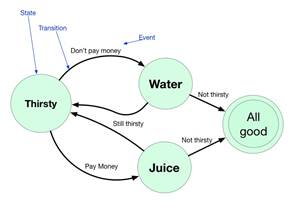Figure 1: A simple state machine where a decision needs to be made.

### 2           Building a state machine

For your third project you will be building a state machine with the simple task of recognizing if a sequence is valid or invalid based on some rules. There are a number of tasks that you will need to satisfy for the requirements: first is that you must use a hash-map to represent your different states and you must read the rules for that said machine using a text file.1                    public class DFA

2                    {

3                    /* hints for your project*/

4                    // you can have more than one final state

5                    private     List <String> finalStates;

6                    // You only have one starting state in a DFA

7                    private     String Start;

8                    // What state are you on now?

9                    private     String currentState;

10                   // Where are you storing your states?

11                   private     HashMap <String,String> stateMap ;

12

13               /*...*/

14               }For this project you will need to read from a text file. You will be building your state machine as you read the input. How you define your input is completely up to you, you can make an object State or you can just represent them as

strings. (I only care that it works)1                         /**

3                         Decides the rules of the state machines.

4                         @var State is the current state

5                         @var stateInput is the input from the sequence

6                         @var State1 is the state resulted from the input

7                         After the states are decided we put them in the stateMap HashMap

8                         */

10                       {

11

12                                                                              String State;

13                                                                              String stateInput;

14                                                                              String transitionState;

15                                                                              // DFARules.txt is just the file name with your rules

16                                                                              // You can give it another name and another address

17                                                                              java.io.File file = new java.io.File("DFARulles.txt");

18                                                                              try {

19                                                                              Scanner input = new Scanner(file);

20                                                                              while (input.hasNext())

21                                                                              {

22                                                                              String cord = input.nextLine();

23                                                                              Scanner Scord = new Scanner (cord);

24                                                                              State= Scord.next();

25                                                                              // populate your state machine

26                                                                              // Hint: Since every machine is

27                                                                              // labled by a number 1-n

28                                                                              // use the key for each map

29                                                                              // the same way you would for a

30                                                                              // point in an x,y grid (2D)

31                                                                              // For example (fromstate "," input)

32                                                                              }

33                                                                              }

34                                                                              catch (FileNotFoundException e)

35                                                                              {

36                                                                              //We are kinda screwed then

37                                                                              System.out.println("No State Machine rules found. "

38                                                                              + "Please look at the instructions and try again.");

39                                                                              }

40

41                                  }### 4           What it should look like

The Java DFA you make will take in two inputs. One input will be from a text file and the other will be in the form of comandline input (string) to test if a sequence satisfies the DFA rules. The text file can be located in the same file as the java files so that you can retain a static address and not have to prompt the user for the rules file. It would be nicer to prompt the user for the file but that is a bit too much to do.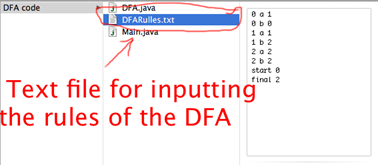Figure 2: An example of what the project should look like in the file being submitted. I don’t want a project!

For simplicity the user would enter the state then the input and the next transition state (all three separated by space) on one line. For stating that a state is the start state the user types “start” before it to hint the program that it is a start state. For the final states the user can type the word “final” before them to indicate that those states are final states. Each rule should be on a separate line otherwise you will have a hard time making this.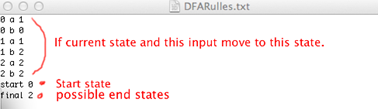Figure 3: An example of a set of rules in a text file.

Then compile the main class preferably in jGRASP and enter a sequence you wish to test without any spaces for example: abba

Is it free to get my assignment evaluated?

Yes. No hidden fees. You pay for the solution only, and all the explanations about how to run it are included in the price. It takes up to 24 hours to get a quote from an expert. In some cases, we can help you faster if an expert is available, but you should always order in advance to avoid the risks. You can place a new order here.

How much does it cost?

The cost depends on many factors: how far away the deadline is, how hard/big the task is, if it is code only or a report, etc. We try to give rough estimates here, but it is just for orientation (in USD):

 Regular homework \$20 - \$150 Advanced homework \$100 - \$300 Group project or a report \$200 - \$500 Mid-term or final project \$200 - \$800 Live exam help \$100 - \$300 Full thesis \$1000 - \$3000

How do I pay?

Credit card or PayPal. You don't need to create/have a Payal account in order to pay by a credit card. Paypal offers you "buyer's protection" in case of any issues.

Why do I need to pay in advance?

We have no way to request money after we send you the solution. PayPal works as a middleman, which protects you in case of any disputes, so you should feel safe paying using PayPal.

Do you do essays?

No, unless it is a data analysis essay or report. This is because essays are very personal and it is easy to see when they are written by another person. This is not the case with math and programming.

Why there are no discounts?

It is because we don't want to lie - in such services no discount can be set in advance because we set the price knowing that there is a discount. For example, if we wanted to ask for \$100, we could tell that the price is \$200 and because you are special, we can do a 50% discount. It is the way all scam websites operate. We set honest prices instead, so there is no need for fake discounts.

Do you do live tutoring?

No, it is simply not how we operate. How often do you meet a great programmer who is also a great speaker? Rarely. It is why we encourage our experts to write down explanations instead of having a live call. It is often enough to get you started - analyzing and running the solutions is a big part of learning.

What happens if I am not satisfied with the solution?

Another expert will review the task, and if your claim is reasonable - we refund the payment and often block the freelancer from our platform. Because we are so harsh with our experts - the ones working with us are very trustworthy to deliver high-quality assignment solutions on time.

## Popular Solved Assignments Like This

Customer Feedback

"Thanks for explanations after the assignment was already completed... Emily is such a nice tutor! "

Order #13073